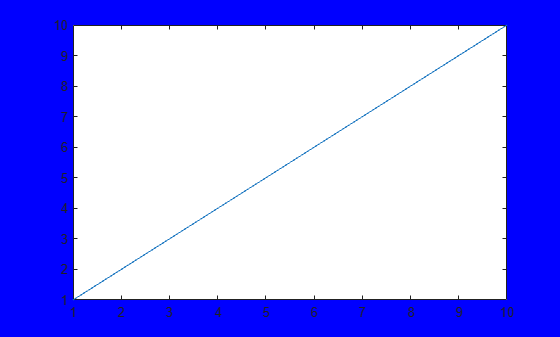# clf

## 语法

``clf``
``clf(fig)``
``clf('reset')``
``clf(fig,'reset')``
``f = clf(___)``

## 说明

``clf` 删除当前图窗中具有可见句柄的所有子级。`

``clf(fig)` 删除指定图窗中具有可见句柄的所有子级。`

``clf('reset')` 删除当前图窗的所有子级，不管其句柄可见性如何。它还将图窗属性重置为默认值，但 `Position`、`Units`、`PaperPosition` 和 `PaperUnits` 属性除外。您也可以使用不带括号的 `clf reset` 命令来重置图窗。`

``clf(fig,'reset')` 删除指定图窗的所有子级并重置其属性。`

``f = clf(___)` 可使用上述任一语法返回图窗。`

## 示例

```x = linspace(0,2*pi); y = sin(x); plot(x,y) f = gcf; f.Color = [0 0.5 0.5];````clf`

```f = clf('reset'); f.Children```
```ans = 0x0 empty GraphicsPlaceholder array. ```

```f1 = figure('Color','b'); plot(1:10)``````f2 = figure; plot((1:10).^2)````clf(f1)`

`clf(f1,'reset')`

```figure('Color','w') peaks```
``` z = 3*(1-x).^2.*exp(-(x.^2) - (y+1).^2) ... - 10*(x/5 - x.^3 - y.^5).*exp(-x.^2-y.^2) ... - 1/3*exp(-(x+1).^2 - y.^2) ````clf reset`

## 提示

• 要清空图窗的内容，您也可以使用图窗窗口的编辑菜单中的清空图窗。使用清空图窗会删除图窗中具有可见句柄的所有子级。